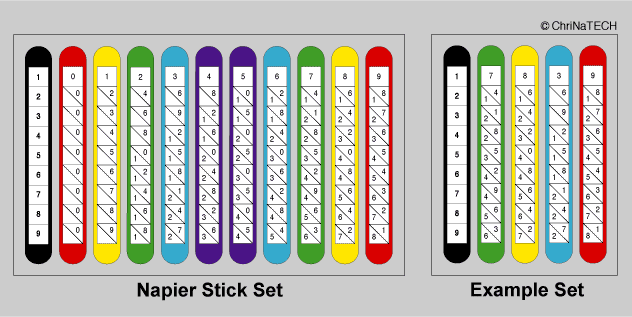NAPIER's BONES Just for fun: Build your own "NAPIER Sticks"``` Historical Remarks: ------------------- It was the Scottish mathematician John Napier (1550-1617), who invented LOGARITHMS to simplify multiplication and division. Based on this concept, the English mathematician William Oughtred (1574-1660) invented the useful SLIDE RULE. J.Napier is also credited with invention of the DECIMAL POINT for the notation of decimal fractions. But he became widely known for his clever multiplication tool, refered as "NAPIER's BONES". Originally made of rectangular wooden pieces, every "bone" shows the (1..9) multiplication-table of the "bone"-number (0..9) in a special way: The 10s-figure is separated by a diagonal from the 1s-figure. To calculate numbers with repeated figures, every 4-sided "bone" of the (10 piece) set carries 4 different figures - except the "index" with the figures 1..9 Here is a home-made version, made out of "Craft Sticks". Two black "index- sticks" with figures (1..9) are 1-sided. The other (e.g. 20) sticks carry on the back side the 9s-Complement of their front side: (0;9) = Red, (1;8) = Yellow, (2;7) = Green, (3;6) = Blue, (4;5) = Violet. H O W T O U S E N A P I E R ' s B O N E S ================================================= See Example Setting: 7839 * 526 ---------------------- 47034 (= 6*7839) 15678 (= 2*7839) 39195 (= 5*7839) ---------------------- 4123314 ======= The problem will be solved as usual (multiplying and writing from right to left) but using the "Napier's Bones" to make it easier! Starting with *6 use line 6 of the "index-bone" in the example setting: index 7 8 3 9 +-------+-------+-------+-------+-------+ | | / | / | / | / | | 6 | 4 / 2 | 4 / 8 | 1 / 8 | 5 / 4 | | | / | / | / | / | +-------+-------+-------+-------+-------+ 4 7 0 3 4 <<<=== ( *6 ) 6*9=54. The 4 (right of the diagonal in column 9) is written down. The carry-over 5 (left of the diagonal in column 9) is added to the 8 right of the diagonal in column 3: (8+5)=13. The 3 is written down and the carry-over 1 is added to the next-left "diagonal-pair" (8+1)+1=10. The 0 is written down and the carry-over 1 is added to the next-left "diagonal- pair" (2+4)+1=7. The 7 is written down. Because there is no carry-over, the remaining 4 is written down. Proceed the same way with *2 (=line 2) and *5 (=line 5) and indent the numbers. Add the columns for the result. In Feb.2015 a gift from my friend WOLF BLUEMICH a SET OF "NAPIER BONES": ==========================================================================RECOMMENDATIONS for a P R O J E C T of "DO-IT-YOURSELF BONES" ... -------------------------------------------------------------------------- Instead of random selected surfaces of "Bone-Numbers", it is easier to find "The Right Bone Number", when a "SYSTEM" is used, eg. ...... the 9s-Complement on opposite sides ! impressum: ************************************************************************** © C.HAMANN http://public.beuth-hochschule.de/~hamann 09/30/05 ```